×#### Thank you for registering.

One of our academic counsellors will contact you within 1 working day.

Click to Chat

1800-1023-196

+91-120-4616500

CART 0

• 0

MY CART (5)

Use Coupon: CART20 and get 20% off on all online Study Material

ITEM
DETAILS
MRP
DISCOUNT
FINAL PRICE
Total Price: Rs.

There are no items in this cart.
Continue Shopping• Complete JEE Main/Advanced Course and Test Series
• OFFERED PRICE: Rs. 15,900
• View Details

```Chapter 9: Trigonometric Ratios of Multiple and Sub Multiple Angles – Exercise 9.3

Trigonometric Ratios of Multiple and Sub Multiple Angles – Exercise 9.3 – Q.1

We have,

sin2 72° - sin260°.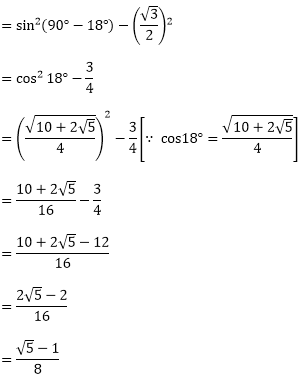Trigonometric Ratios of Multiple and Sub Multiple Angles – Exercise 9.3 – Q.2

L.H.S = sin2 24° - sin26°

= sin(24 + 6) sin(24 - 6) [ ∵  sin(A + B)sin(A - B) = sin2A - sin2B]

= sin 30°sin 18°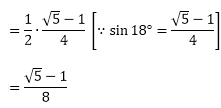= RHS

Trigonometric Ratios of Multiple and Sub Multiple Angles – Exercise 9.3 – Q.3

L.H.S = sin242° - cos278°

= sin2(90 - 48) - cos2(90 - 12)

= cos248° - sin212°

= cos(48 + 12).cos(48 - 12) [∵ cos(A + B).cos(A - B) = cos2A - sin2B]

= cos60°.cos36°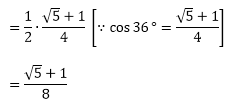= RHS

Trigonometric Ratios of Multiple and Sub Multiple Angles – Exercise 9.3 – Q.4

L.H.S = cos78°.cos42°.cos36°= RHS

Trigonometric Ratios of Multiple and Sub Multiple Angles – Exercise 9.3 – Q.5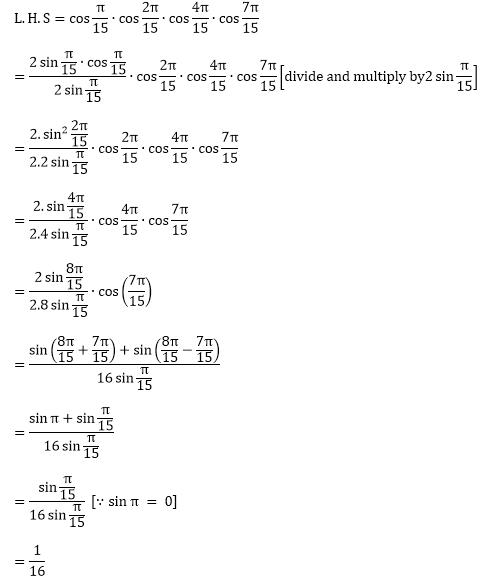= RHS

Trigonometric Ratios of Multiple and Sub Multiple Angles – Exercise 9.3 – Q.6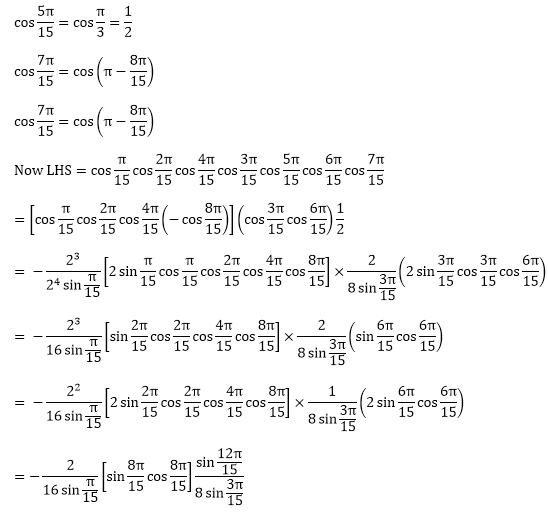Trigonometric Ratios of Multiple and Sub Multiple Angles – Exercise 9.3 – Q.7

L.H.S = cos 6°.cos 42°.cos 66°.cos 78°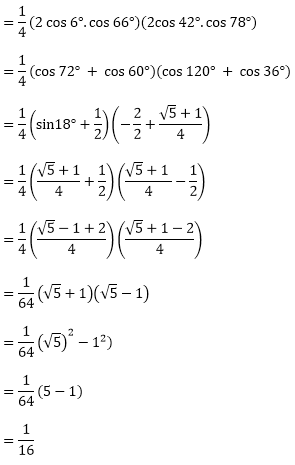= RHS

Trigonometric Ratios of Multiple and Sub Multiple Angles – Exercise 9.3 – Q.8

L.H.S = sin 6°.sin 42°.sin 66°.sin 78°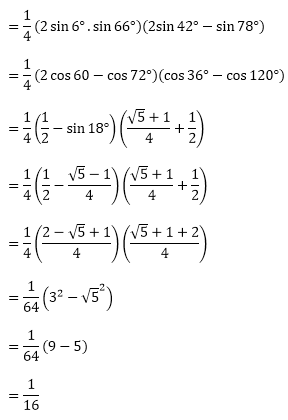= RHS

Trigonometric Ratios of Multiple and Sub Multiple Angles – Exercise 9.3 – Q.9

L.H.S = cos 36°.cos 42°.cos 60°.cos 78°= RHS

Trigonometric Ratios of Multiple and Sub Multiple Angles – Exercise 9.3 – Q.10

sin36°.sin72°.sin108°.sin144°

[∵ sin144°= sin (180° - 36°) = sin36° and sin108° = sin (180° - 78°) = sin72°]

= sin 36°.sin 72°.sin 72°. sin 36°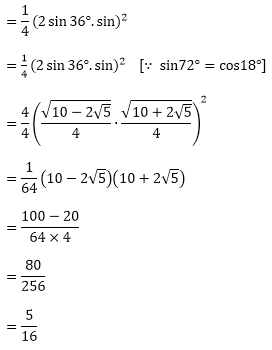= RHS
```### Course Features

• 728 Video Lectures
• Revision Notes
• Previous Year Papers
• Mind Map
• Study Planner
• NCERT Solutions
• Discussion Forum
• Test paper with Video Solution